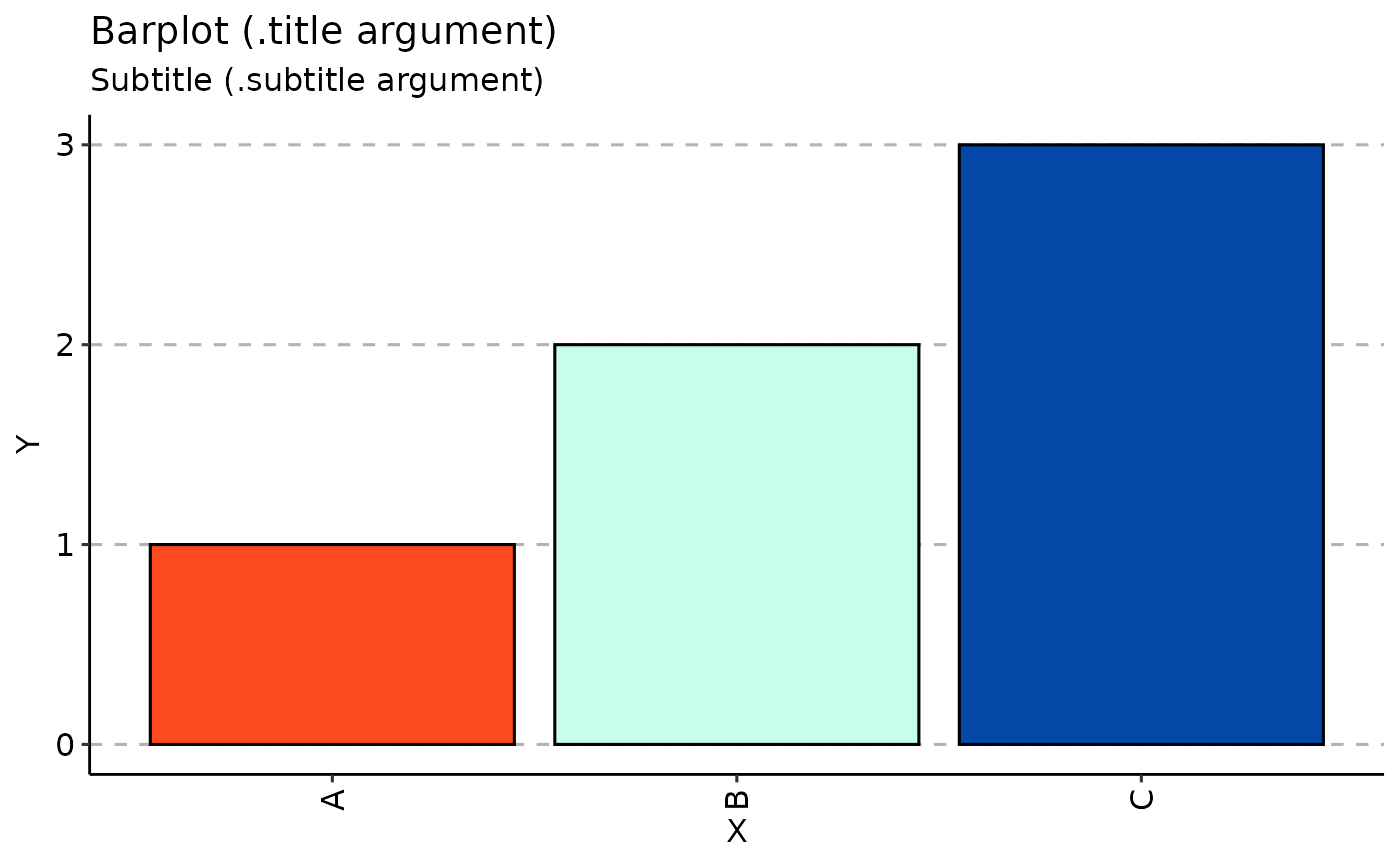Bar plots

vis_bar(
.data,
.by = NA,
.meta = NA,
.errorbars = c(0.025, 0.975),
.errorbars.off = FALSE,
.stack = FALSE,
.points = TRUE,
.test = TRUE,
.signif.label.size = 3.5,
.errorbar.width = 0.2,
.defgroupby = "Sample",
.grouping.var = "Group",
.labs = c("X", "Y"),
.title = "Barplot (.title argument)",
.subtitle = "Subtitle (.subtitle argument)",
.legend = NA,
.leg.title = "Legend (.leg.title argument)",
.legend.pos = "right",
.rotate_x = 90
)

## Arguments

.data

Data to visualise.

.by

Pass NA if you want to plot samples without grouping.

You can pass a character vector with one or several column names from ".meta" to group your data before plotting. In this case you should provide ".meta".

You can pass a character vector that exactly matches the number of samples in your data, each value should correspond to a sample's property. It will be used to group data based on the values provided. Note that in this case you should pass NA to ".meta".

.meta

A metadata object. An R dataframe with sample names and their properties, such as age, serostatus or hla.

.errorbars

A numeric vector of length two with quantiles for error bars on sectors. Disabled if ".errorbars.off" is TRUE.

.errorbars.off

If TRUE then plot CI bars for distances between each group. Disabled if no group passed to the ".by" argument.

.stack

If TRUE and .errorbars.off is TRUE then plot stacked bar plots for each Group or Sample

.points

A logical value defining whether points will be visualised or not.

.test

A logical vector whether statistical tests should be applied. See "Details" for more information.

.signif.label.size

An integer value defining the size of text for p-value.

.errorbar.width

Numeric. Width for error bars.

.defgroupby

A name for the column with sample names.

.grouping.var

A name for the column to group by.

.labs

A character vector of length two specifying names for x-axis and y-axis.

.title

The text for the plot's title.

.subtitle

The text for the plot's subtitle.

.legend

If TRUE then displays a legend, otherwise removes legend from the plot.

.leg.title

The text for the plots's legend. Provide NULL to remove the legend's title completely.

.legend.pos

Positions of the legend: either "top", "bottom", "left" or "right".

.rotate_x

How much the x tick text should be rotated? In angles.

## Value

A ggplot2 object.

## Examples

vis_bar(data.frame(Sample = c("A", "B", "C"), Value = c(1, 2, 3)))